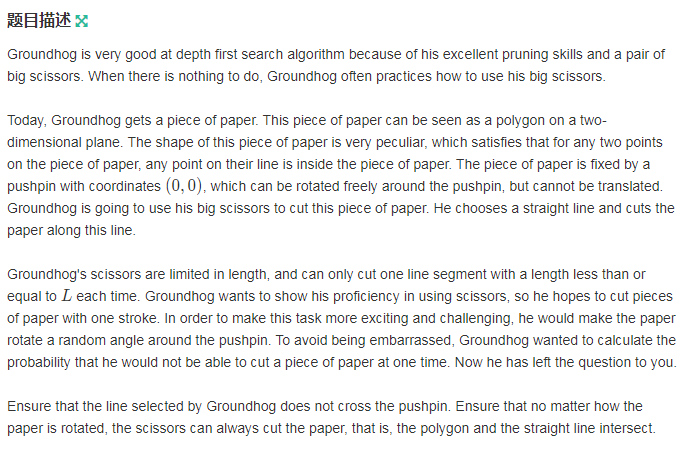# Description• 题目给你一个凸多边形，可以绕原点随便转，剪刀固定方向，限制长度，求可以剪开的概率

# Solution

• 这是一种巧妙的暴力做法
• 转多边形很麻烦，我们相对地想到转剪刀方向
• 我们每次把剪刀转一点点
• “一点点”来自精度要求 1 0 − 4 10^{-4} 2 π 3 ∗ 1 0 5    r a d \cfrac{2\pi}{3*10^5}\,\,rad 绰绰有余
• 算出剪刀沿此方向需要剪的距离，与 L L 比较
• 如果超过 L L 说明剪不开
#include <bits/stdc++.h>
const int N=1e5+10;
const double P=acos(0.0)*2.0,eps=1e-8;
using namespace std;
int n;double d,L;
int sgn(double x){
return (fabs(x)<eps)?0:(x<0?-1:1);}
struct Point{

double x,y;
Point(){
}
Point(double _x,double _y){
x=_x,y=_y;}
Point operator +(Point a){
return Point(x+a.x,y+a.y);}//向量加
Point operator -(Point a){
return Point(x-a.x,y-a.y);}//减
double operator *(Point a){
return x*a.x+y*a.y;}//点积
Point operator %(double a){
return Point(x*a,y*a);}//数乘（伸缩）Scaling
double operator ^(Point a){
return x*a.y-y*a.x;}//叉积
}v[N<<2];
struct Line{
Point s,e;Line(){
}Line(Point _s,Point _e){
s=_s,e=_e;}}ln;
Point rotate(Point c,double a){
return Point(c.x*cos(a)-c.y*sin(a),c.x*sin(a)+c.y*cos(a));}
Point LIL(Line a,Line b){
return a.s+(a.e-a.s)%(((b.e-b.s)^(b.s-a.s))/((b.e-b.s)^(a.e-a.s)));}
//Line Intersects Line,直线交直线
double displ(Point a,Line b){

Point v1=b.e-b.s,v2=a-b.s;
return fabs(v1^v2)/sqrt(v1.x*v1.x+v1.y*v1.y);
}int main(){

scanf("%d",&n);
for(int i=1;i<=n;i++)
scanf("%lf%lf",&v[i].x,&v[i].y),v[i+n]=v[i],v[i+2*n]=v[i],v[i+3*n]=v[i];
scanf("%lf%lf%lf%lf%lf",&L,&ln.s.x,&ln.s.y,&ln.e.x,&ln.e.y);
d=displ(Point(0,0),ln);//剪刀到原点的距离
Point p0(0.0,0.0);
int cnt=0,tot=3e5,l=1,r=1;
for(int i=0;i<tot;++i){

double a=i*2*P/tot;
Point cur=Point(cos(a),sin(a))%d;//单位圆定向,d定长
Point vv=rotate(cur,P/2);//剪刀与距离成90角
Point nxt=cur+vv;//中间量
Point p1,p2;
Line s0=Line(cur,nxt);//真正的剪刀方向
while(true){

int o1=sgn((nxt-cur)^(v[l]-nxt));
int o2=sgn((nxt-cur)^(v[l+1]-nxt));
if(o1*o2==1){
++l;continue;}
p1=LIL(s0,Line(v[l],v[l+1]));//往一边找到与多边形相交的点
break;
}r=max(r,l+1);
while(true){

int o1=sgn((nxt-cur)^(v[r]-nxt));
int o2=sgn((nxt-cur)^(v[r+1]-nxt));
if(o1*o2==1){
++r;continue;}
p2=LIL(s0,Line(v[r],v[r+1]));//往另一边找到与多边形相交的点
break;
}Point p=p1-p2;//剪刀剪开需要剪过的向量
double res=sqrt(p.x*p.x+p.y*p.y);//|p|
if(sgn(L-res)<0) ++cnt;
}printf("%.4lf\n",1.0*cnt/tot);
}


### Fabric 1.0源代码分析(18) Ledger（账本）_尹成的博客-程序员秘密

# Fabric 1.0源代码笔记 之 Ledger（账本）## 1、Ledger概述Ledger，即账本数据库。Fabric账本中有四种数据库，idStore（ledgerID数据库）、blkstorage（block文件存储）、statedb（状态数据库）、historydb（历史数据库）。其中idStore、historydb使用leveldb实现，statedb可选择使用leveldb或c...

### boot mybatis mysql_SpringBoot+MyBatis+Mysql 详细示例_葎茜的博客-程序员秘密

SpringBoot与MyBatis整合，底层数据库为mysql的使用示例项目下载链接：https://github.com/DFX339/bootdemo.git新建maven项目，web项目，项目名为 bootdemo项目结构目录如下:还有个pom.xml文件没有在截图里面项目需要编写的文件主要有：项目启动类：Application.java ServletInitializer.jav...

### 让Android模拟器飞一会，模拟器的速度终于可以快过真机啦！（转）_安卓模拟器性能比真机强_进击的魔法师的博客-程序员秘密

PS：有的人安装过程中遇到这个问题this computer meets the reauirements for HAXM,but....这个问题应该是CPU可能默认没有开Vt，所以得去bios开了再说。进了bios找到virtual technology选项，选择enable即可。android的模拟器一直以来是它的一大败笔，启动需要很长时间，运

### 系统程序员成长计划-容器与算法(二)（上）_李先静的博客-程序员秘密

系统程序员成长计划-容器与算法(二)（上）  转载时请注明出处和作者联系方式文章出处：http://www.limodev.cn/blog作者联系方式：李先静 容器用来存储数据，算法用来处理数据。容器有多种，算法的种类更多，两者的组合数目就数不胜数了。如果同样的算法要为每种容器都写一遍，写的时候单调不说，维护起来也很困难。所以我们一直在寻找让算法独立